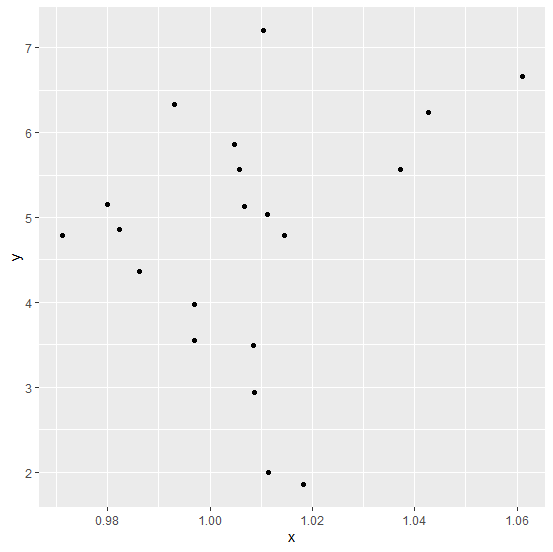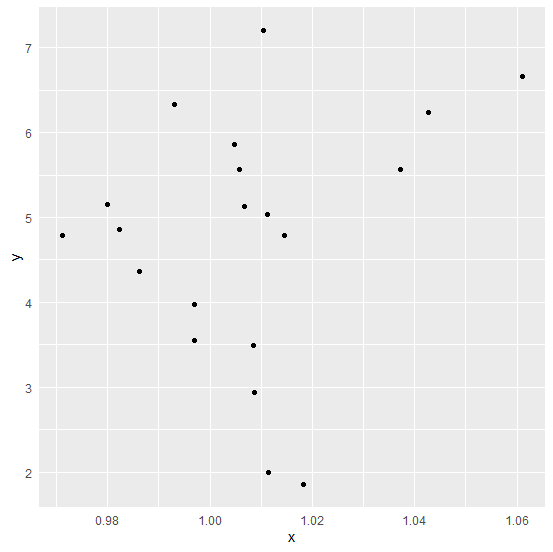# How to remove ticks in a plot created by using gglot2 in R?

In a plot created by using ggplot2, the axes values are generated with tick marks such as representing X-axis labels from 1 to 10 and Y-axis labels from 10 to 1 but we can get rid of this tick marks by using theme function. If we want to create a plot without ticks then we just need to add the following code to the plot code.

theme(axis.ticks.x=element_blank(),axis.ticks.y=element_blank())

## Example

Consider the below data frame.

Live Demo

> set.seed(321)
> x<-rnorm(20,1,0.025)
> y<-rnorm(20,5,1.35)
> df<-data.frame(x,y)
> df

## Output

x y
1 1.0426226 6.238295
2 0.9821990 4.855467
3 0.9930504 6.334253
4 0.9970088 3.552478
5 0.9969010 3.976679
6 1.0067046 5.128251
7 1.0181710 1.853243
8 1.0058284 5.563647
9 1.0084778 3.487558
10 0.9862021 4.359176
11 1.0086925 2.933944
12 1.0371148 5.561215
13 1.0047081 5.856167
14 1.0610815 6.661644
15 0.9711640 4.791339
16 0.9798832 5.154628
17 1.0114017 1.994545
18 1.0105083 7.208317
19 1.0144396 4.784917
20 1.0111589 5.038161

Loading ggplot2 package and creating a point chart between x and y.

> library(ggplot2)
> ggplot(df,aes(x,y))+geom_point()

## OutputCreating point chart using ggplot2 without ticks on the axes.

## Example

> ggplot(df,aes(x,y))+geom_point()+theme(axis.ticks.x=element_blank(),axis.ticks.y=element_blank())

## Output